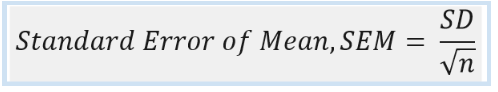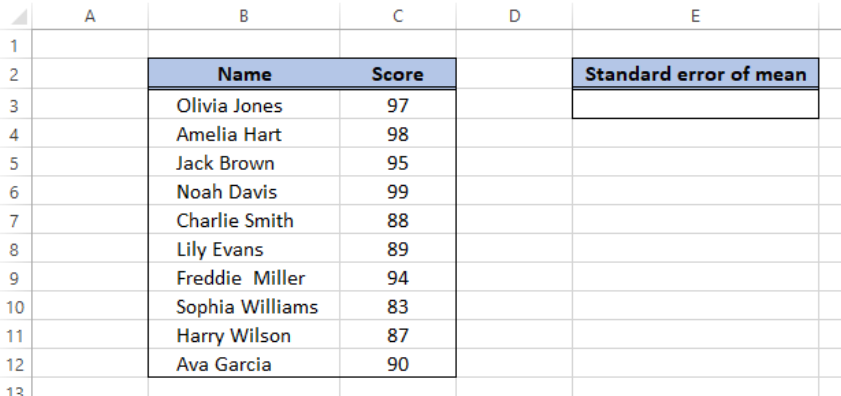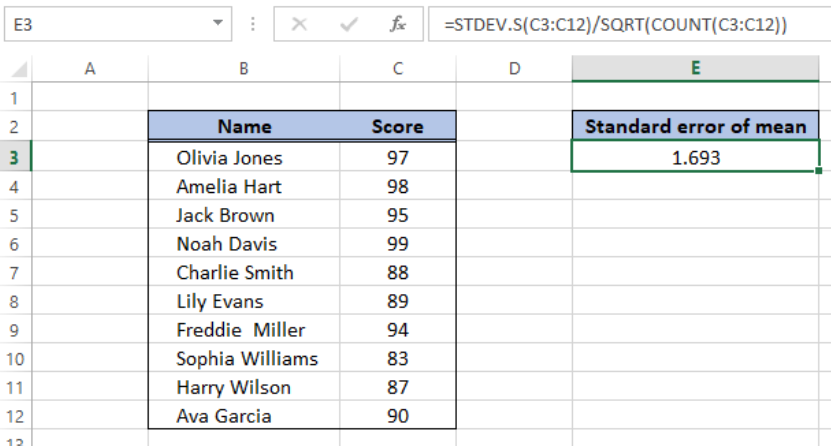Get instant live expert help with Excel or Google Sheets“My Excelchat expert helped me in less than 20 minutes, saving me what would have been 5 hours of work!”

#### Post your problem and you'll get expert help in seconds

Your message must be at least 40 characters
Our professional experts are available now. Your privacy is guaranteed.

# Calculate standard error of mean (SEM) in Excel

The Standard Error of Mean, also known as SEM is another measure of variability of data.  It is an estimate of the deviation of a sample mean from the population mean.  SEM is not as popular as standard deviation, and it is sometimes just referred to as “standard error”.

Its formula is the quotient of standard deviation and the square root of sample size.

## Formula for SEMFigure 1. Standard error of mean formula

There is no built-in function that directly computes for the standard error of mean.  We can calculate the standard error of mean by using the functions STDEV.S, SQRT and COUNT.

Standard error of mean formula:

`= STDEV.S(sample)/SQRT(COUNT(sample))`

Parameters:

• STDEV.S function returns the standard deviation of a sample
• SQRT function returns the square root of a number
• COUNT function returns the number of data points in a sample

## How to find standard error

By using the above formula, we can calculate the standard error of mean through these steps:

• Prepare data in worksheetFigure 2.  Sample data for standard error of mean

• Select cell E3 and enter the formula for SEM:

`=STDEV.S(C3:C12)/SQRT(COUNT(C3:C12))`Figure 3.  Final result:  Standard error of mean

As a result, the standard error of mean is 1.693, as calculated in cell E3.

## Instant Connection to an Excel Expert

Most of the time, the problem you will need to solve will be more complex than a simple application of a formula or function. If you want to save hours of research and frustration, try our live Excelchat service! Our Excel Experts are available 24/7 to answer any Excel question you may have. We guarantee a connection within 30 seconds and a customized solution within 20 minutes.